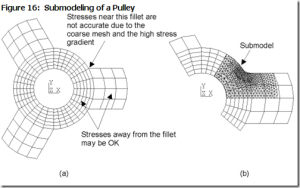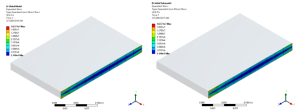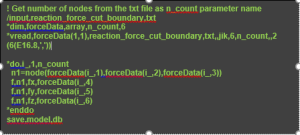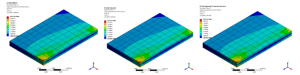# Force Based SubmodelingEven with today’s increased computing power and optimized software, submodeling is a powerful technique to perform analysis on detailed regions of assemblies. Recent versions of ANSYS Mechanical software have easy to use implementation for submodeling, where one can perform cut boundary interpolations all in Workbench environment, without the need of writing good old APDL code.

Submodeling in ANSYS Mechanical (and APDL) is based on interpolation displacements from global level to submodel level at cut boundaries. This approach comes with the assumption that the stiffness of the submodel region does not differ much in stiffness from the global model. This assumption holds most of the time. However, there are cases where one would like to apply structural loads from global model, rather than the displacements. This is especially a requirement when the stiffness of the model is reduced in the modeled sub region. The technique can be also called “Force Based Submodeling”. Below is a naïve implementation of force based submodeling for solid-to-solid case.Need: We would like to apply the force being carried by supports in a submodel, rather than performing a displacement based submodel. The design of the support is changed such that it will invalidate global displacement results. Therefore we propose a way to extract reaction forces at cut boundaries rather than displacements in classical submodeling sense.

Algorithm:

1. Perform global analysis with coarse mesh
2. Generate a submodel(a.k.a. initial submodel) analysis, with exact same geometry of global analysis with coarse mesh. This will allow one to get reaction forces where cut-boundary displacements are applied
3. Extract reaction forces on cut-boundary faces
1. Can extract reaction forces on whole surfaces and apply them as remote forces with deformable boundary option
2. Can extract reaction forces on each node and apply in final submodel by ensuring same mesh on cut-boundaries between initial submodel and final submodel
4. Generate a submodel (a.k.a. final submodel) with updated support design
5. Apply reaction forces calculated from initial submodel
6. Solve for final submodel and post-process

# Example Case#1

I have built a simple model to test the above mentioned concept:Fig. Boundary conditions (a) Global model (b) Initial submodel

When I compare the stress at fixed support surface I get identical Von Mises stress distribution.Fig. Von Mises stress at fixed support surface (a) Global model (b) Initial submodel

An APDL code is developed to extract nodal reaction forces from the initial sub model as follows:The text file generated by APDL code based on “initial submodel”, is then manually copied to solver directory of the “final submodel”. This process can be automized by saving the file to “user files” directory instead.

The content of the reaction force text file is then read into “final submodel” by the following APDL code:Comparing the stresses and displacements there is perfect match between global, initial and final submodel models.Fig. Von Mises Stress (a) Global Model (b) Initial submodel (c) Final submodelFig. Total displacements (a) Global Model (b) Initial submodel (c) Final submodel

By Can Ozcan